# Pendulum Lab

## Introduction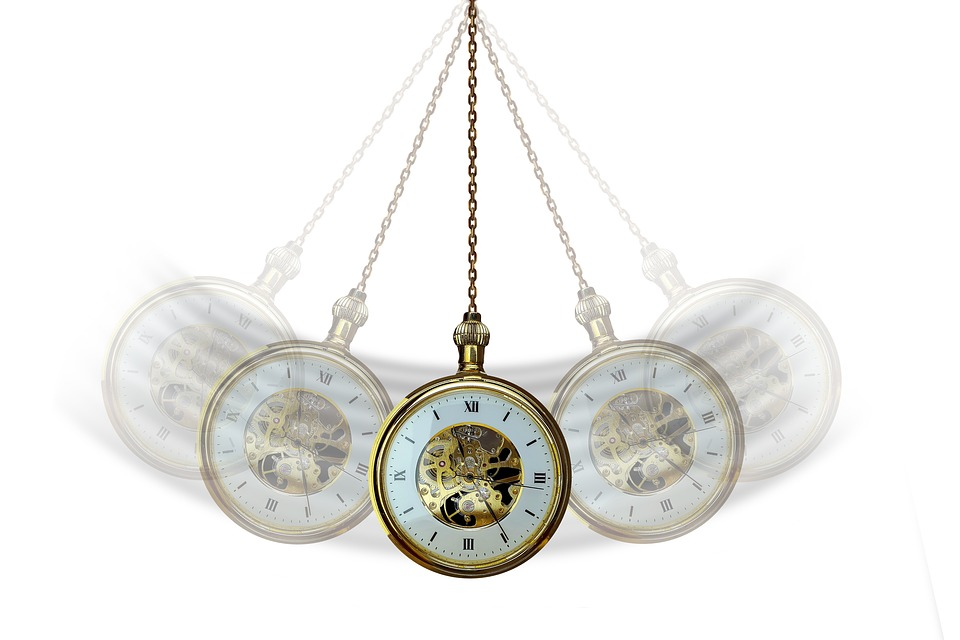What makes a pendulum work?

If you were building a clock how would you figure out what size and mass of pendulum you need?

First we need to do some experiements to see what affects the speed (time) of a pendulum. The most obvious ones to look at are:

• Mass
• Length

Does a heavier weight on the end make the pendulum faster or slower?

What about making the pendulum longer, what happens?

## 1) Period vs Length

1. Wrap 1m of string around the end of the stick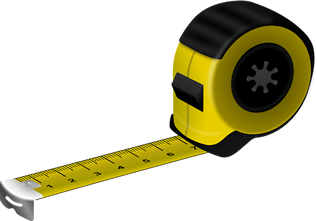2. Hold the stick on the table with about 20-30cm sitcking out past the edge
3. Tie 4 metal weights on the end of a piece of string
4. Adjust the length of string to 60cm
(Measure from the middle of the weights to the stick)
5. Raise the mass to about 45 degrees
6. Time how long 10 full periods (swings) take
7. Repeat for each length shown in the table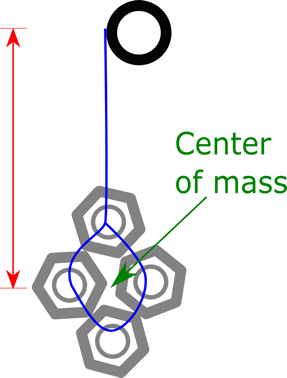Length (m) 10 Periods (s) 1 T (Period) (s)
0.60
0.50
0.40
0.30
0.20

### Question

What effect does the pendulum's length have on its period?

## 2) Period vs Mass

1. Tie 8 metal weights on the end of the string
2.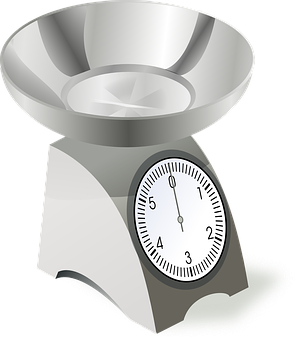Measure and record the mass on the end of the string
3. Adjust the length of string to 60cm
(Measure from the middle of the weights to the stick)
4. Raise the mass to about 45 degrees
5. Measure and record how long 10 full periods (swings) take
6. Repeat for masses of 4, 2, and 1 wieghts
# Weights Length (m) Mass (g) 10 Periods (s) 1 T (Period) (s)
8 0.60
4 0.60
2 0.60
1 0.60

### Question

What effect does the pendulum's mass have on its period?

## 3) DIY Clock

###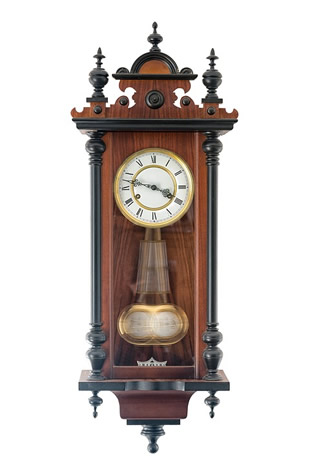Challenge

Can you build a pendulum with a period of exactly 1 second?

How long does it need to be?

Can you make it keep time for 1 minute?

(If you are VERY good at algebra, you could solve the equation below, for L, to figure it out too)

## 4) Calculated vs Measured

Now we need to look at how our measurments compare to theory using maths.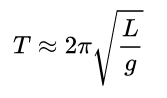T = Period (in seconds)
L = length (in meters)
g = gravity (9.81m/s2)

### Example calculation for a scientific calculator:

60cm = 0.6m

2 x π x √ (0.6 ÷ 9.81) =

### Example calculation for a simple calculator: (We must follow the order of operations manually)

60cm = 0.6m

0.6 ÷ 9.81 = √ x 2 x π =

### Calculate the theorical period for all the lengths you measured:

Length (m) T Period (s)
Measured
Experimental
T Period (s)
Calculated
Theorectical
% Error
0.60
0.50
0.40
0.30
0.20

Scroll to Top